Transcripted Summary

Many times, in programming, our code has to deal with arrays where we don't know exactly what's inside of them.

And if we don't know what values are inside, and where exactly they are, we're unable to reference the exact element location when we need to. For this, there are ways to search an array, and we'll talk about two common ways to do this.

The first approach is to use a sequential search algorithm. The sequential search algorithm searches every element in the array until it finds the value that it's looking for. Or, until it arrives at the end of the array.

This algorithm is okay for small arrays but it's inefficient for arrays with more than several thousand elements.

Another search algorithm is the binary search. For the binary search the array must first be sorted.

So, in the case of our lottery ticket array, the elements would need to be in ascending order from least to greatest. Then you will write code that will begin the search, by checking the element in the middle of the array, to see if it's equal, greater than, or less than the value that you're searching for.

• If it's greater than, then there's no need to search the entire second half of the array. Because we know, that everything over there will be greater than as well. And the element you're searching for is definitely not over there.

• Likewise, if the middle number is less than the value you're searching for, then there's no need to search the entire first half of the array.

• And if the value is equal, you're lucky and you're done.

If it's not equal you've still eliminated half of the array where you know the value is not, and then you continue with another iteration.

Each iteration continues like this — eliminating half of the array for what needs to be searched, until you find the value, or you search the entire array.

This is a much quicker approach than the sequential search. And this algorithm is one that you should definitely know as it's often brought up in tech interviews.

You can code all of this by hand. But if you need to use this in real life, simply use this handy util method called `Arrays.binarySearch()`.If you purchased a lottery ticket that had duplicate numbers, you'd be pretty upset. And right now, our program doesn't handle this case. So, let's update our class to ensure that there are no duplicates, and we'll do that by searching the array.

And I'll show you both the sequential and binary searches.

Let’s look at our `generateNumbers()` method.

``````public static int[] generateNumbers(){

int[] ticket = new int[LENGTH];
Random random = new Random();

for(int i=0; i< LENGTH; i++){
ticket[i] = random.nextInt(MAX_TICKET_NUMBER) + 1;
}

return ticket;
}
``````

We're generating a random number, and then we're just storing inside of this array. There is no guarantee that this number will be different every time this loop iterates. So, we want to get rid of possible duplications.

To do this, before we add this number to the array, we need to first search the array to see if that number already exists.

We're going to come inside of this loop and instead of assigning the random number directly to the array, let's put this in its own variable.

``````public static int[] generateNumbers(){

int[] ticket = new int[LENGTH];
Random random = new Random();

for(int i=0; i< LENGTH; i++){
int randomNumber = random.nextInt(MAX_TICKET_NUMBER) + 1;
}

return ticket;
}
``````

And now we want to search the array. We want to at least search one time. And if the number is already in the array, then we'll need to generate another number.

Let's put this inside of a `do while` loop.

``````for(int i=0; i< LENGTH; i++){
int randomNumber;

/*
Generate random number then search to make sure it doesn't
already exist in the array. If it does, regenerate and search again.
*/
do{
randomNumber = random.nextInt(MAX_TICKET_NUMBER) + 1;
}while();
}
``````

And now we're going to want to search.

So, in this `while`, we're going to make a call to a new method that we're going to create called `search()`.

And we're going to give it our array and the number that was generated.

``````for(int i=0; i< LENGTH; i++){

int randomNumber;

/*
Generate random number then search to make sure it doesn't
already exist in the array. If it does, regenerate and search again.
*/
do{
randomNumber = random.nextInt(MAX_TICKET_NUMBER) + 1;
}while(search(ticket, randomNumber));
}
``````

We're going to keep looping until it finds a number that is not already inside of the array. So `search()` should return a true or false. True if it actually finds the number in the array, meaning it's a duplicate. And so, we'll loop until it's not a duplicate.

Let's go ahead and make this `search` method.

``````public static boolean search(int[] array, int numberToSearchFor){

}
``````

Let me show you another type of comment in Java.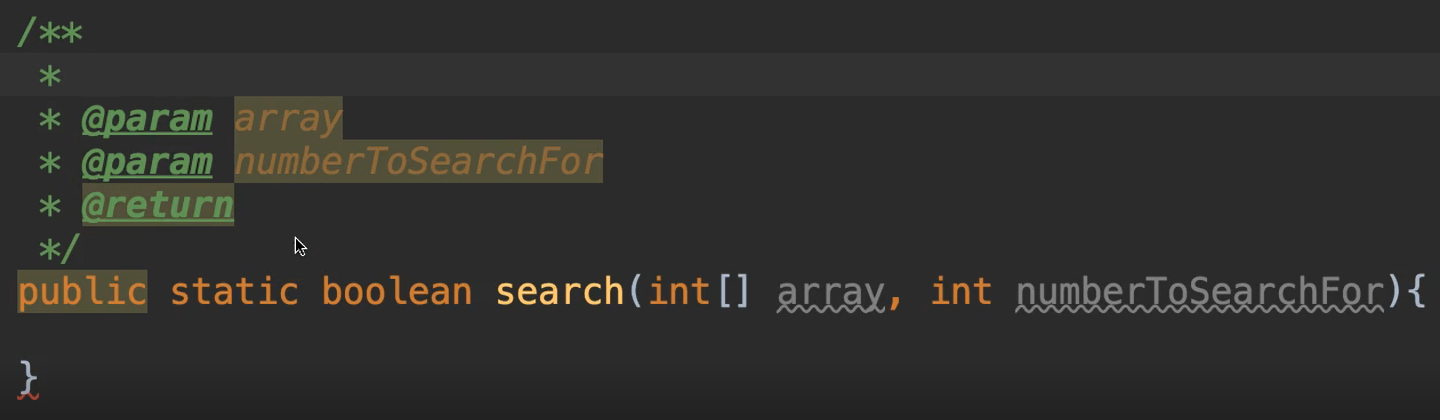If you do the slash and then two asterisks — `//**` — this turns green. And when I pressed enter, all of this was pre-populated.

This is a special kind of comment called a Javadoc.

A Javadoc allows the programmer to give a description of how this method works. So if anyone wants to call this method, they can see the Javadoc from where they're calling from. This gives them some helpful information about how to use our method.

We know this method does a search, but it doesn't really say how it works exactly. We can put a little information in the Javadoc to say what kind of search we're doing.

``````/**
* Does a sequential search on the array to find a value
* @param array Array to search through
* @param numberToSearchFor Value to search for
* @return true if found, false if not
*/
public static boolean search(int[] array, int numberToSearchFor){

}
``````

And we keep it really simple. You put as much as you want. And then you describe what the parameters are and what the return will be. This helps us without having to read through the code to see what it does. We can just have this nice little description.

Okay, so inside of this method, we're going to loop through the array and look at each element of the array. And if that element's value is equal to the number that we're searching for, we're going to return true.

So, I'm going to show you a new type of loop that's called the enhanced for loop.

``````/*
This is called an enhanced loop.
It iterates through 'array' and
each time assigns the current element to 'value'
*/
for(int value : array){

}
``````

The enhanced for loop iterates through the array and each time assigns the current element to `value`.

So, it says `for`, and instead of doing the `int[i] = 0` and all of this stuff, we can just declare something. We'll say `int value` and then we use a colon and say, what do you want to loop through?

So, the 1st value will be the value of the first element in the array.

And we can say if `value`is equal to the number that we're supposed to be searching for, go ahead and return true.

``````for(int value : array){
if(value == numberToSearchFor){
return true;
}
}
``````

So, this will get outside of the loop and outside of the method really. It's just going to go straight to the `return`.

Now we don't put the `else` inside of this loop. Why not?

Because we're looping through this. If we look at the very 1st value and it's not equal, then it would come to the `else`. And if we were to do an `else return false;` in here, then it would exit this loop after only looking at the first element of the array. It never even searched the rest of the array.

So that's not what we want. We want to go through, loop the entire array. If it finds it, `return true`. If it doesn't find it, do nothing. Go to the next one. Keep looping.

Once it gets outside of the loop that means the entire array was searched and the value was not found.

``````public static boolean search(int[] array, int numberToSearchFor){

/*
This is called an enhanced loop.
It iterates through 'array' and
each time assigns the current element to 'value'
*/
for(int value : array){
if(value == numberToSearchFor){
return true;
}
}

/*
If we've reached this point, then the entire array was searched
*/
return false;
}
``````

So, if we get to this point, then we can say `return false`.

Okay, so that gives us the sequential loop. And that solves the problem.

I'm just going to show you the binary search really quickly, even though we're not going to use it.

I just want to show it to you in action.

I was going to call this new method `search` and just make it an overloaded method, but I cannot, because it's going to take the same parameter list. And so, it won't be unique.

So, I named this `binarySearch`

``````public static boolean binarySearch(int[] array, int numberToSearchFor){

}
``````

Now remember the steps of the binary search. The array must be sorted first.

``````public static boolean binarySearch(int[] array, int numberToSearchFor){

//Array must be sorted first
}
``````

And we can do that with this `Arrays` class I was telling you about.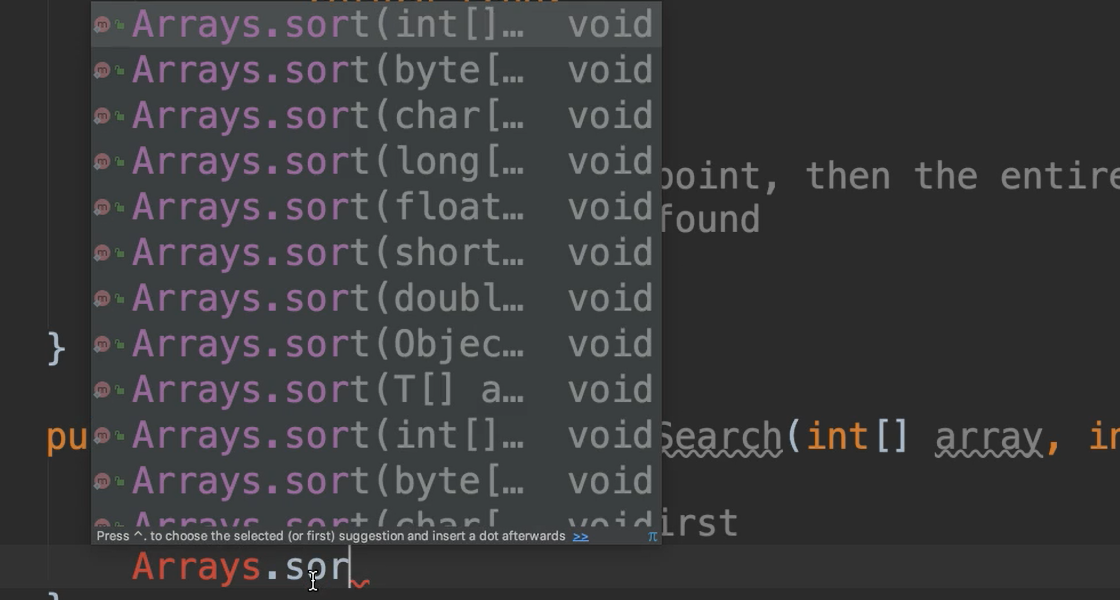It's a utility class. It has all kinds of nice things in here.

One of the things that it has is this `sort`.

``````public static boolean binarySearch(int[] array, int numberToSearchFor){

//Array must be sorted first
Arrays.sort(array);
}
``````

`sort` will put all of the elements of `array` in ascending order.

Then we are going to call `Arrays.binarySearch()`:

``````public static boolean binarySearch(int[] array, int numberToSearchFor){

//Array must be sorted first
Arrays.sort(array);
Arrays.binarySearch(array, numberToSearchFor);
}
``````

We're going to pass it our `array`, and then we're going to pass it our `numberToSearchFor`.

So, if we hover over this and click the command key, it'll give us some information for the signature of this `binarySearch`.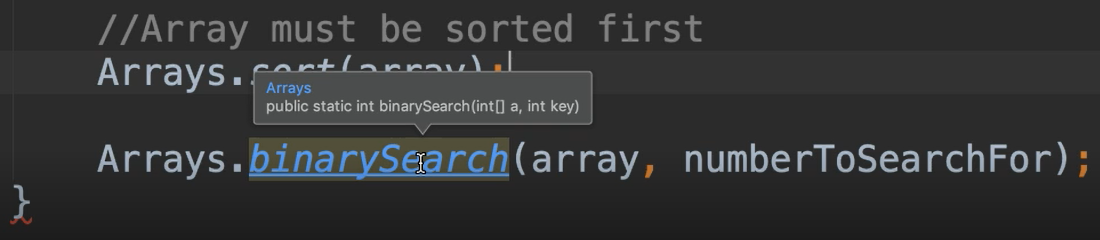It says that this is going to return an integer back and I'm going to just click this. If I click this I can see that Javadoc that I told you about. And it shows me what's going on. So, it says that, it searches the specified array of integers for a specified value…

What I really want to know is what is being returned exactly.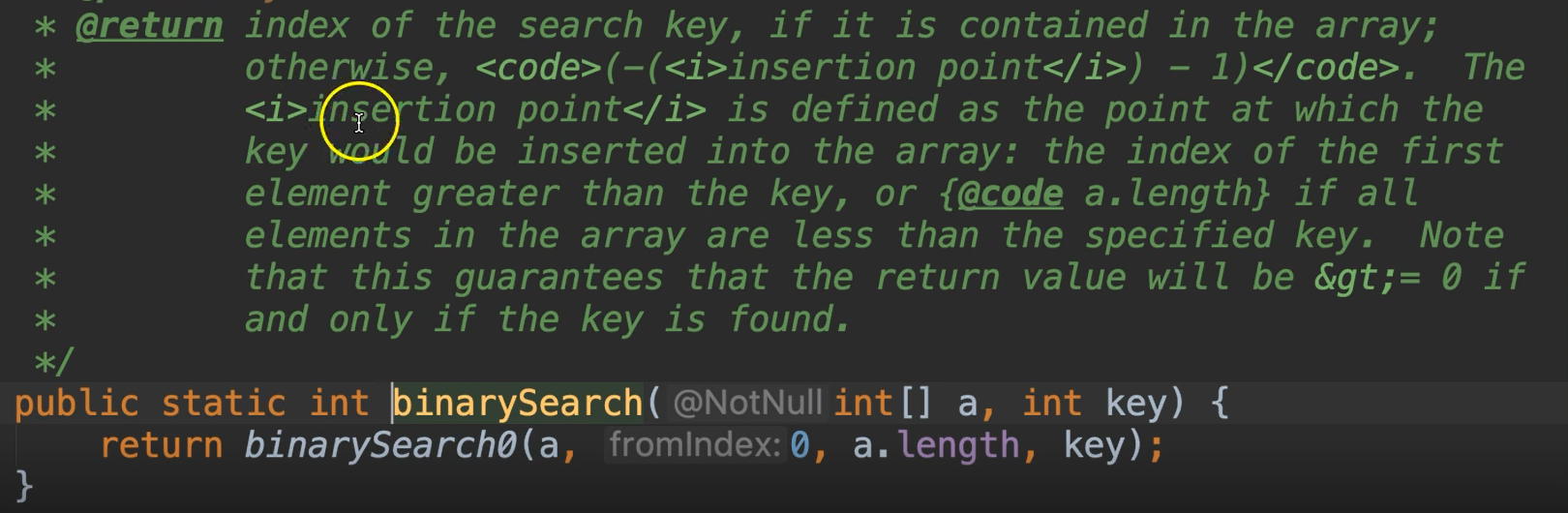So, this is going to return an integer greater than or equal to 0 if and only if the key is found. So great.

We'll go ahead and assign this an integer; we'll call it “index”.

And if the `index` is greater than or equal to 0, we know that it was found. We'll go ahead and return true, else return false.

``````int index = Arrays.binarySearch(array, numberToSearchFor);
if(index >= 0){
return true;
}
else return false;
``````

Okay, so that's the binary search, but we're not using that in our program. I just did that to show you how it works.

We're actually going to use is the first `search()` method.

Okay, let's go ahead and run this and just make sure it still works.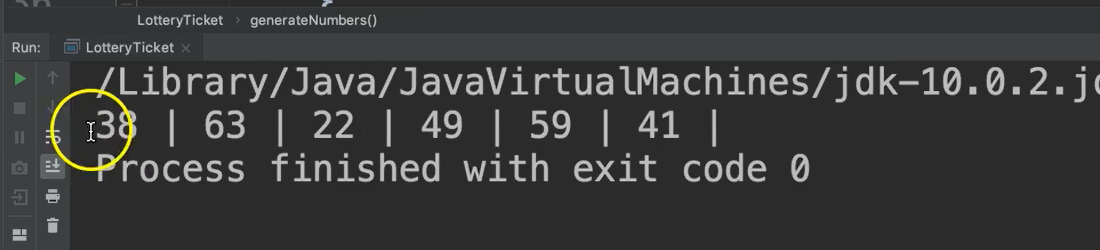Great. So, all of our numbers are unique.

Now, even though our numbers are unique, it was hard to see that at a glance because they're not in order. When we get our lottery tickets, they're typically printed in ascending order.

Let's go ahead update our program once more so that I can show you about sorting arrays.

If we go to our `main` method, we generate the ticket. And before we print the ticket let's just sort this array.

``````public static void main(String[] args){

int[] ticket = generateNumbers();
Arrays.sort(ticket);
printTicket(ticket);
}
``````

Great, let’s run it!

### # LotteryTicket.java

``````package chapter7;

import java.util.Arrays;
import java.util.Random;

public class LotteryTicket {

private static final int LENGTH = 6;
private static final int MAX_TICKET_NUMBER = 69;

public static void main(String[] args){

int[] ticket = generateNumbers();
Arrays.sort(ticket);
printTicket(ticket);
}

public static int[] generateNumbers(){

int[] ticket = new int[LENGTH];

Random random = new Random();

for(int i=0; i< LENGTH; i++){
int randomNumber;

/*
Generate random number then search to make sure it doesn't
already exist in the array. If it does, regenerate and search again.
*/
do{
randomNumber = random.nextInt(MAX_TICKET_NUMBER) + 1;
}while(search(ticket, randomNumber));

//Number is unique if we get here. Add it to the array.
ticket[i] = randomNumber;
}

return ticket;
}

/**
* Does a sequential search on the array to find a value
* @param array Array to search through
* @param numberToSearchFor Value to search for
* @return true if found, false if not
*/
public static boolean search(int[] array, int numberToSearchFor){

/*
This is called an enhanced loop.
It iterates through 'array' and
each time assigns the current element to 'value'
*/
for(int value : array){
if(value == numberToSearchFor){
return true;
}
}

/*
If we've reached this point, then the entire array was searched
*/
return false;
}

public static boolean binarySearch(int[] array, int numberToSearchFor){

//Array must be sorted first
Arrays.sort(array);

int index = Arrays.binarySearch(array, numberToSearchFor);
if(index >= 0){
return true;
}
else return false;
}

public static void printTicket(int ticket[]){
for(int i=0; i<LENGTH; i++){
System.out.print(ticket[i] + " | ");
}
}
}
``````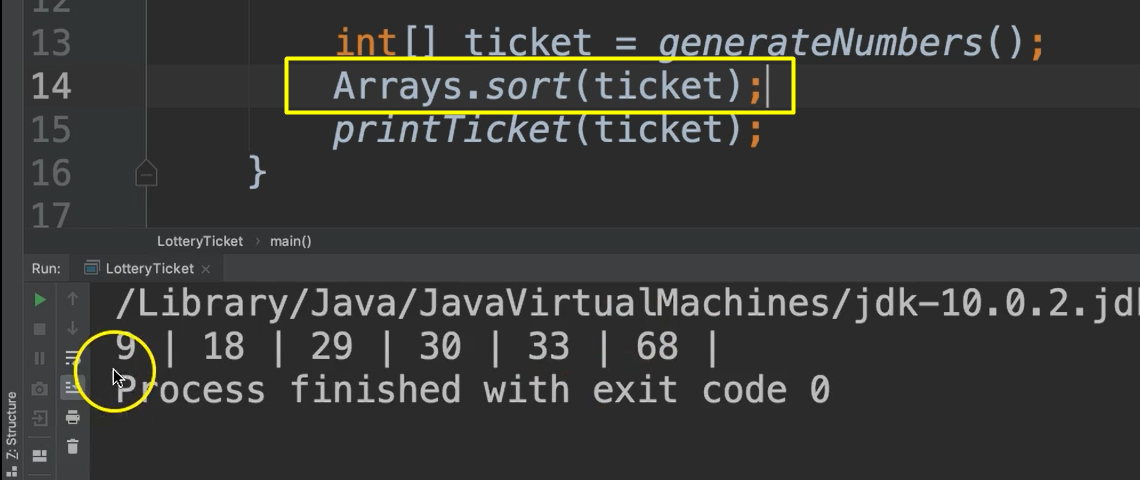Nice. It's in ascending order.